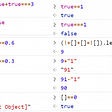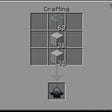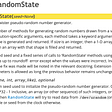# 2D range sum, Kadane’s algorithm and maximum subarray sum no more than k

## 2D range sum based

We can use brute force solution with 2D range sum.

The complexity is O(row ^ 2 * col ^ 2)

`int cusum;class Solution {public:    int maxSumSubmatrix(vector<vector<int>>& matrix, int k) {        const int m = matrix.size(), n = matrix.size();        //using vector will get TLE        //vector<vector<int>> cusum(m + 1, vector<int>(n + 1, 0));        memset(cusum, 0, sizeof cusum);        for (int i = 1; i <= m; ++i)            for (int j = 1; j <= n; ++j)                cusum[i][j] = cusum[i-1][j] + cusum[i][j-1] - cusum[i-1][j-1] + matrix[i-1][j-1];        int ret = INT_MIN, thisret = 0;         for (int i = 1; i <= m; ++i)            for (int j = 1; j <= n; ++j)                for (int r = i; r <= m; ++r)                    for (int c = j; c <= n; ++c){                        thisret = cusum[r][c] - cusum[i-1][c] - cusum[r][j-1] + cusum[i-1][j-1];                        if (thisret <= k) ret = max(ret, thisret);                     }        return ret;    }};`

## An improved version based on 

We are going to use 2D Kadane’s algorithm and set to solve this problem.

`// O(ROW^2 * COL * log COL)class Solution {public:    int max_no_larger_k(vector<int> colPrefix, int k) {        // https://www.quora.com/Given-an-array-of-integers-A-and-an-integer-k-find-a-subarray-that-contains-the-largest-sum-subject-to-a-constraint-that-the-sum-is-less-than-k        // O(COL log COL)        set<int> s;        int ret = INT_MIN, cusum = 0;        s.insert(cusum);  s.insert(cusum);        for (auto & x: colPrefix){            cusum += x;            // cusum - cusum_old <= k            // cusum_old >= cusum - k            int cusum_old = cusum - k;            auto it = s.lower_bound(cusum_old);            if (it != s.end()) ret = max(ret, cusum - (*it));            s.insert(cusum);        }        return ret;    }    int maxSumSubmatrix(vector<vector<int>>& matrix, int k) {        const int ROW = matrix.size(), COL = matrix.size();        // the prefix sum per col from row1 to row2        int ret = INT_MIN;        // reference https://www.youtube.com/watch?v=yCQN096CwWM        // solve it along the row instead of along the column        // O(ROW^2 * COL)        for (int row1 = 0; row1 <  ROW; ++ row1){            vector<int> row1ToRow2_ColPrefix(COL, 0);            for (int row2 = row1; row2 < ROW; ++ row2){                // update the column direction prefixsum                for (int j = 0; j < COL; ++j)row1ToRow2_ColPrefix[j] += matrix[row2][j];                 int thisret = max_no_larger_k(row1ToRow2_ColPrefix, k);                ret = max(ret, thisret);            }        }        return ret;    }};`

# Reference

--

--

--

## More from Jimmy Shen

Data Scientist/MLE/SWE @takemobi

Love podcasts or audiobooks? Learn on the go with our new app.

## REACT NATIVE NUGGET- ScrollView Vs FlatList## React Props and TypeScript## Comparison with “==” and “===” is just a difference of type checking in JavaScript, think again?## Building an app in Vue JS (webpack, axios, bootstrap 4, reddit, and infinite scrolling in vanilla…## Important of react## Custom JavaScript in Cognos Analytics II— Date Prompts and Datasets## Bedrock Killer Whale Vehicle Addon## Jimmy Shen

Data Scientist/MLE/SWE @takemobi

## Interview With our Newest Part-Timer## An intro about evolutionary algorithms## How np.random.seed and np.random.randn works## Making an AI for a coding interview question